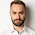## Sunday, 19 February 2012

### Calender program in C

//this is a calender program which accepts date in the format dd mm yyyy and prints day on this date
#include<stdio.h>
#include<conio.h>
int calmd(int n);
void main()
{

int d,m,y,a,x,md;
printf("Enter the date in format dd mm yyyy\n");
scanf("%d %d %d", &d, &m, &y);
if((m==2&&y%4==0&&d>29)||(m==2&&y%4!=0&&d>28))
printf("Wrong date !\n Welcome to amancybertricks.blogspot.com");
else if(((m==4||m==6||m==9||m==11)&&(d>30))||((m==1||m==3||m==5||m==7||m==8||m==10||m==12)&&(d>31)))
printf("Wrong date !\n Welcome to amancybertricks.blogspot.com");

else
{
printf("Welcome to amancybertricks.blogspot.com\n It is ");
md = calmd(m);
a = y*365+(y-1)/4+md+d;
x = a%7;
if((y%4==0)&&(m>2))
x=x+1;
switch(x)
{
case 1:
printf("Saturday");
break;
case 2:
printf("Sunday");
break;
case 3:
printf("Monday");
break;
case 4:
printf("Tuesday");
break;
case 5:
printf("wednessday");
break;
case 6:
printf("Thrusday");
break;
default:
printf("Friday");
break;
}
}
getch();
}
int calmd(int n)
//this program is Written by aman kumar singh
{ int md;
if(n==1)
md=0;
else if(n==2)
md=31;
else if(n==3)
md=59;
else if(n==4)
md=90;
else if(n==5)
md=120;
else if(n==6)
md=151;
else if(n==7)
md=181;
else if(n==8)
md=212;
else if(n==9)
md=243;
else if(n==10)
md=273;
else if(n==11)
md=304;
else
md=334;
return md;
}

1.2.3.